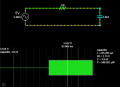# RC Voltage Divider ---> Output Voltage =/= to Calculated

#### imzack

Joined Nov 3, 2010
73
In the below circuit, I would expect to see 2.5v peak AC across the Capacitor; but according to the sim, I am seeing 3.5v. Any thoughts on why?Resistor = 12k
C = 1/(2*pi*f*C)
= 1/ (2*pi* 3.4kHz * 3.9nF)
= 12k

Since the impedance of the resistor and capacitor are both at 12k, I would expect the output voltage to be 1/2 its input voltage...

Vout = Vin *( 12 / (12 + 12))
= Vin * (12/24)
= Vin* 1/2

Where am I going wrong?

Thanks!

#### ericgibbs

Joined Jan 29, 2010
17,145
hi,
Is the Voltage source 2.5 Vpeak or 2.5Vrms value, as 2.5Vrms *1.414 = ~3.5Vpk
E

•bertus

#### crutschow

Joined Mar 14, 2008
31,506
Where am I going wrong?
You are neglecting the phase-shift caused by the capacitor which affects the attenuation, thus you must use complex (vector) arithmetic for the magnitude calculation.
(Have you studied AC circuits? If not start here.)
The attenuation is then Xc/√(R²+Xc²) or 12k / 16.97k = .707 (the -3dB point) where Xc is the capacitor impedance at the frequency of interest.
And 5V * .707 = 3.5V, the value you observed.

Last edited:
•TeeKay6 and imzack